Courses

# Short & Long Answer Question (Part-1) - Thermal Properties of Matter Class 11 Notes | EduRev

## Class 11 : Short & Long Answer Question (Part-1) - Thermal Properties of Matter Class 11 Notes | EduRev

The document Short & Long Answer Question (Part-1) - Thermal Properties of Matter Class 11 Notes | EduRev is a part of the Class 11 Course Physics Class 11.
All you need of Class 11 at this link: Class 11

Q.1 Is temperature a macroscopic or microscopic concept?

Answer: Temperature is macroscopic concept. It is related to the average kinetic energy of a large number of molecules forming a system. It is not possible to define the temperature for a single molecule.
Q.2 What is dynamical theory of heat?

Answer: According to dynamical theory, the amount of heat possessed by a body is equal to the total kinetic energy of its molecules and its temperature is proportional to the average kinetic energy of its molecules.
Q.3 Two thermometers are constructed in the same way except that one has a spherical bulb and the other an elongated cylindrical bulb. Which of the two will respond quickly to temperature changes?

Answer:  A cylindrical bulb has a greater surface area than a spherical bulb of the same volume. Hence the thermometer with elongated bulb will respond to temperature changes more quickly than the one with a spherical bulb.
Q.4 Why a clinical thermometer should not be sterilized by boiling?

Answer: The range of clinical thermometer is usually from 95F to 110°F and the boiling point of water is 212 F. So on sterilization by boiling, the capillary of thermometer will burst due to thermal expansion of mercury in the capillary.
Q.5 Why should a thermometer bulb have a small heat capacity?

Answer: The thermometer bulb having small heat capacity will absorb less heat from the body whose temperature is to be measured. Hence the temperature of that body will practically remain unchanged.
Q.6 What do you mean by triple point of water? Why it is unique?

Answer: It is the temperature at which the three phases of water: ice, liquid water and water vapour are equally stable and exist simultaneously. It is unique because it occurs at specific temperature (= 273.16 K) and a specific pressure of about 0.46 cm of Hg column.
Q.7 Why are gas thermometers are more sensitive than mercury thermometers?

Answer: The coefficient of expansion of a gas is very large as compared to the coefficient of expansion of mercury. For the same temperature range, a gas would undergo a much larger change in volume as compared to mercury.

Q.8 Can the temperature of a body be negative on the kelvin scale?

Answer: No. This is because the absolute zero on the kelvin scale is the minimum possible temperature.
Q.9 Mercury boils at 357 C How can then a mercury thermometer be used to measure temperature up to 500C?

Answer: The space above the mercury is filled with nitrogen. This increases the boiling point of mercury and raises the upper limit of the mercury thermometer.
Q.10 Why the temperatures above 1200 C cannot be measured accurately by a platinum resistance thermometer?

Answer: This is because platinum begins to evaporate above 1200C
Q.11 Why is a constant volume gas thermometer preferred as a standard thermometer than a constant pressure gas thermometer?

Answer: This is because the changes in pressure can be measured with greater accuracy than changes in volume.
Q.12 A mercury thermometer is transferred from melting ice to a hot liquid. The mercury rises 0.9 of the distance between lower and upper fixed points. What is the temperature of the liquid inC? What in F?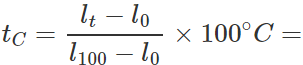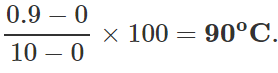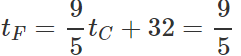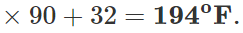Q.13 The readings of air thermometer at 0C and 100 C are 50 cm and 75 cm of mercury column respectively. What is the temperature at which its reading is 80 cm of Hg column?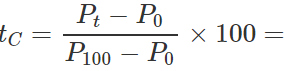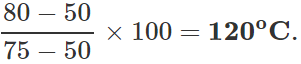Q.14 Two bodies at different temperatures T1 and T2 , if brought in thermal contact do not necessarily settle at the mean temperature (T1+T2)/2. Why?

Answer: The two bodies may have different masses and different materials i.e., they may have different thermal capacities. In case the two bodies have equal thermal capacities they would settle at the mean temperature (T1+T2)/2

Q.15 A body at higher temperature contains more heat, comment.

Answer: The statement is not always true. The heat content of a body depends upon its mass, specific heat and temperature.
Q.16 Two hollow glass balls are connected by  a tube, which has a pellet of mercury in the middle. Can the temperature of the surrounding air be determined from the position of the drop?

Answer: Yes. If the tube is held vertically, the position of the pellet will change with any change in the temperature of the surrounding air. This can be used as a thermometer.
Q.17 Do all solids expand on heating? If not, give an example.

Answer: No. Camphor contracts on heating.
Q.18 Why does a solid expand on heating?

Answer: The average distance between the positions of equilibrium of the atoms of a solid increases with an increase in temperature which results in the thermal expansion of a solid.
Q.19 Is the temperature coefficient always positive?

Answer: No. Temperature coefficient α is positive for metals and alloys and negative for semiconductors and insulators.
Q.20 The diameters of steel rods A and B having the same length are 2 cm and 4 cm respectively. They are heated through 100∘ C. What is the ratio of increase of length of A to that of B?

Answer: 1:1.This is because the increase in length does not depend on the diameter of the steel rod.

Q.21 The difference between lengths of a certain brass rod and that of a steel rod is claimed to be constant at all temperatures. Is this possible?

Answer: Yes. This is possible when the lengths of the rods are in inverse ratio of their coefficients of linear expansion. For the difference in the lengths of the two rods to remain same,
Δl1=Δl2 or l1α1ΔT=l2α2ΔT   or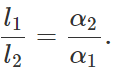Q.22 Why a small gap is left between the iron rails of railway tracks?

Answer: If no gap is left between the iron rails, the rails may bend due to expansion in summer and the train may get derailed.

Q.23 Why are loops provided in long metal pipes used for carrying oil and any other liquid over long distances?

Answer: The loops in metal pipes are provided in order to avoid the strain that would develop in the pipes when the temperature changes and hence the pressure of liquids in them changes.
Q.24 Pendulum clocks generally run fast in winter and slow in summer. Why?

Answer: The time period of a simple pendulum is given by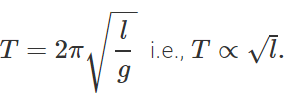. In winter, l decreases with the fall in temperature, so T decreases and clocks run fast. In summer increases with the increase in temperature, so T increases and clock runs slow.

Q.25 Why is invar used in making a clock pendulum?

Answer: Invar, which is an alloy of nickel and steel, has extremely small temperature coefficient of expansion and so the length of a pendulum made of invar does not change appreciably during summer and winter seasons and hence the clock gives almost correct time.
Q.26 Why must telephone or power lines necessarily slag a little?

Answer: The sag is allowed for contraction in winter. If no sag is allowed, the wire may snap in extremely cold weather.
Q.27 A brass disc fits snugly in a hole in a steel plate. Should we heat or cool the system to loosen the disc from the hole?

Answer: α for brass is greater than that for steel. On cooling, the disc shrinks to a greater extent than the hole, and hence the disc would get loosened.
Q.28 A tightened glass stopper can be taken out easily by pouring hot water around the neck of the bottle. Why?

Answer: The neck expands but not the stopper due to poor conductivity of glass. Thus the stopper can be taken out easily.

Q.29 A long cylindrical vessel having linear coefficient of expansion α is filled with a liquid up to a certain level. On heating, it is observed that the length of the liquid in the cylinder remains the same. What is the volume coefficient of expansion of the liquid?

Answer: Since the level of liquid remains the same, therefore, the volume coefficient of expansion of the liquid is the same as the volume coefficient of expansion of the cylinder. So, the volume coefficient of expansion of the liquid is 3α.
Q.30  Thick bottomed drinking glasses frequently crack if hot water is poured into them. Why?

Answer: Glass is a bad conductor of heat. It does not pass down the heat quickly to the lower surface. Different layers of the bottom are at different temperatures and expand differently. This causes breakage of the glass at the bottom.
Q.31 Two identical rectangular strips of copper, and the other of steel are riveted to form a bimetallic strip. What will happen on heating?

Answer: Since α for copper is more than a for steel, hence on heating, the bi-metallic strip will bend in such a way that the copper strip remains on outer or convex side.
Q.32 Why iron rims are heated red hot before being put on the cart wheels?

Answer: The iron ring to be put on the rim of a cart wheel is always of slightly smaller diameter than that of the wheel. When the iron ring is heated to become red hot, it expands and slips on to the wheel easily. When it is cooled, it contracts and grips the wheel firmly.
Q.33 In riveting boiler plates, red hot rivets are used. Why?

Answer: Red hot rivets are used so that on cooling, the grip becomes firm and steam-tight.
Q.34 How does the diameter of the opening in the cast iron plate of a kitchen stove change, when the stove is heated?

Answer: The opening in the stove is circular. But its diameter undergoes linear expansion on heating. The increase in diameter is given by: ΔD=αDΔT.
Q.35 A metal ball is heated through a certain temperature. Out of mass, radius, surface area and volume, which will undergo largest  percentage increase and which one the least?

Answer: The mass of the ball will not change. Its volume (4/3 πr3) will undergo largest percentage increase while the percentage increase in radius will be minimum.
Q.36 Explain why a beaker filled with water at 4C overflows if the temperature is decreased or increased?

Answer: It is because of the anomalous expansion of water. Water has a maximum density at 4C. Therefore, water expands whether it is heated above 4C or cooled below 4C.
Q.37 A block of wood is floating on water at 0C with a certain volume V above the level of water. The temperature of water is gradually increased from 0C to 8C. How does the volume V change with the change of temperature?

Answer: The density of water increases from 0C to 4C and decreases from 4C to 8C. So, V will increase till the temperature of water reaches 4C and then it will go on decreasing.
Q.38 Is J a physical quantity?

Answer: No. J is not a physical quantity. It is a conversion factor.
Q.39 Why is J called a conversion factor?

Answer: Because it helps us to convert work measured in terms of joules into heat expressed in calories or vice-versa.
Q.40 A thermos bottle containing water is vigorously shaken. What will be the effect on the temperature of water?

Answer: The temperature of the water will increase slightly. This is because some of the work done against opposing viscous force will be converted into heat.
Q.41 When we rub our hands, they are warmed but only to a certain maximum temperature. Why?

Answer: The work done in rubbing is converted into heat. But after some time when the temperature of the hands is raised, the total heat produced goes into the atmosphere.
Q.42 There is a slight temperature difference between the water fall at the top and the bottom. Why?

Answer: The potential energy of water at the top of the fall gets converted into heat kinetic energy at the bottom of the fall. When water hits the ground, a part of its kinetic energy gets converted into heat which increases its temperature slightly.
Q.43 Why do the brake drums of a car get heated, when the car moves down a hill at a constant speed?

Answer: As the car moves down a hill at constant speed, its kinetic energy does not change. But its gravitational potential energy constantly decreases, which gets converted into heat and so the brake drums get heated.

Q.44 Can a given amount of mechanical energy be completely converted into heat?

Answer: Yes, a given amount of mechanical work can be completely converted into heat. This is because whole of the mechanical energy can be absorbed by the molecules of a system in the form of their kinetic energy which gets converted into heat.

Q.45 A match stick can be lighted by rubbing it against a rough surface. Why?

Answer: When the match stick is rubbed against a rough surface, work is done against friction. This work done appears as heat and lights up the match stick.

Q.46 If an electric fan be switched in a closed room, will the air of the room be cooled? If not, why do we feel cold?

Answer: The air will not be cooled. In fact, it will get heated up due to the increase in the speed of its molecules. We feel cold due to faster evaporation of sweat.
Q.47 Give an example of a system in which no heat is transferred to or from a system but the temperature of the system changes.

Answer: When a paddle-wheel arrangement is worked while dipping in water, the temperature of water increases without any addition of heat.
Q.48 What is the difference between the specific heat and the molar specific heat?

Answer: The specific heat is the heat capacity per unit mass whereas the molar specific heat is the heat capacity per mole.
Q.49 Why water is preferred to any other liquid in the hot water bottles?

Answer: Water is preferred to any other liquid in the hot water bottles because due to high specific heat it does not cool fast. Also for the given mass of water, the amount of heat contained is higher and it can provide more warmth as compared to any other liquid.
Q.50 The coolant used in a nuclear reactor should have high specific heat. Why?

Answer: The purpose of a coolant is to absorb maximum heat with least rise in its own temperature. This is possible only if specific heat is high because Q=mcΔT. For a given value of m and Q the rise in temperature ΔT will be small if c is large. This will prevent different parts of the nuclear reactor from getting too hot.

Offer running on EduRev: Apply code STAYHOME200 to get INR 200 off on our premium plan EduRev Infinity!

,

,

,

,

,

,

,

,

,

,

,

,

,

,

,

,

,

,

,

,

,

;